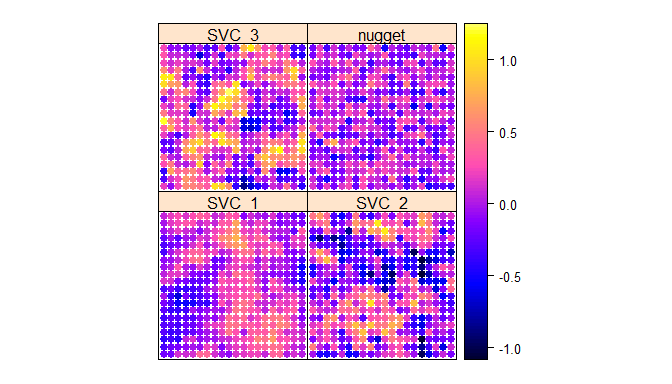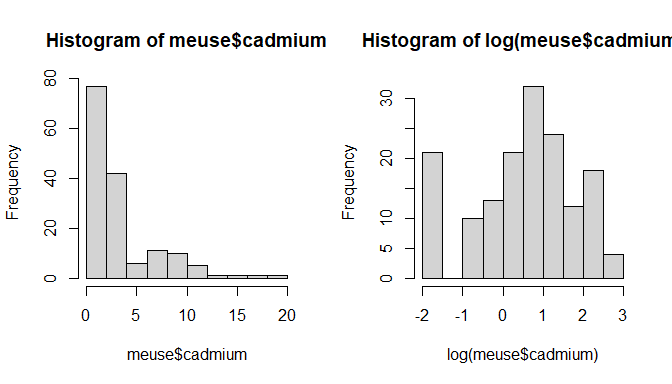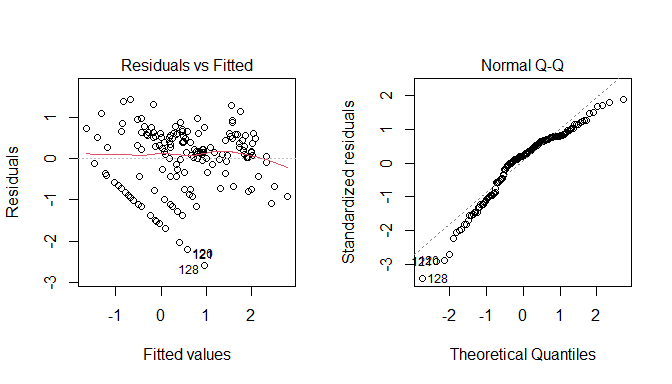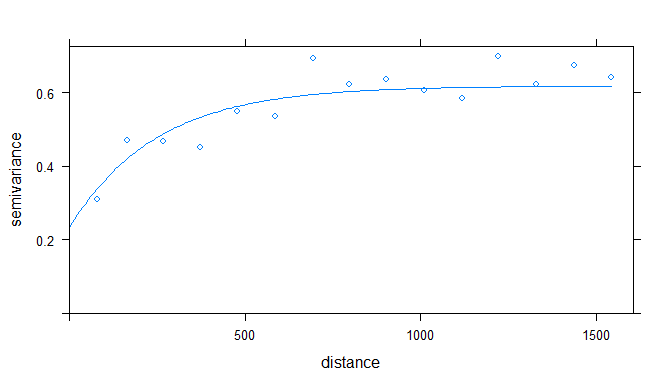# varycoef: An R Package to Model Spatially Varying Coefficients

## Introduction

With the release of the R package varycoef on CRAN (see) we enable you to analyze your spatial data and in a simple, yet versatile way. The underlying idea are spatially varying coefficients, short SVC, which have been studied over the last decades. These models are based on the linear regression model and are therefore very easy to interpret. Due to their design, SVC models offer a high flexibility. These two properties allow for better understanding of the data, gaining new insights and, ultimately, better predictive performance.

In this blog post, we will show what SVC models are and how to define them. Afterwards, we give a short and illustrative example on how to apply these tools in varycoef using the well known data set meuse from the package sp.

Disclaimer This analysis is meant to be a show case of what is possible using the package varycoef, not to write the most rigorous statistical analysis of a data set.

But first, let us start with the set up and let me show you where to find help:

# install from CRAN
# install.packages("varycoef")

# attach package
library(varycoef)

# general package help file
help("varycoef")

# vignette for detailed information on how to use varycoef
vignette("example", package = "varycoef")
## Warning: vignette 'example' not found

There is also a version of varycoef on Github from which you can get the latest devel version.

### Preliminaries

Before we start, we want to give some prerequisites in order to understand the analysis below. Beside a classical linear regression model, we require the knowledge of geostatistics and tools thereof, like:

• what is the advantage of geostatistics?
• two-dimensional Gaussian processes, i.e. Gaussian random fields
• covariance functions and how the range and variance parameter influence them
• maximum likelihood estimation

### What are Spatially Varying Coefficient Models?

SVC models are a generalization of the classical linear regression model. In matrix notation with a response vector $$\mathbf y$$, a data matrix $$\mathbf X$$, and a vector $$\boldsymbol \varepsilon$$ containing the errors, a linear regression model is defined as

$\mathbf y = \mathbf X \boldsymbol \beta + \boldsymbol \varepsilon.$

In geostatistics, the error term usually is called the nugget. SVC models are introduced through a random effect $$\boldsymbol \eta(s)$$ that is depending on the location $$s$$. The underlying model takes the form

$\mathbf y = \mathbf X \boldsymbol \beta + \mathbf W \boldsymbol \eta (s) + \boldsymbol \varepsilon$ where $$\mathbf W$$ parses the covariates to the random effect $$\boldsymbol \eta(s)$$. Specifically, each SVC is defined by a Gaussian process, more specifically a Gaussian random field (GRF), with mean zero and some stationary covariance function. This function usually depends on some marginal variance (sill) and a range. An example of 3 SVC, i.e. 3 GRFs, and the nugget $$\boldsymbol \varepsilon$$ on a regular grid is given in the figure below.

# setting seed
set.seed(123)
# number of SVC
p <- 3
# sqrt of total number of observations
m <- 20
# covariance parameters
(pars <- data.frame(var = c(0.1, 0.2, 0.3),
scale = c(0.3, 0.1, 0.2)))
##   var scale
## 1 0.1   0.3
## 2 0.2   0.1
## 3 0.3   0.2
nugget.var <- 0.05

# function to sample SVCs
sp.SVC <- fullSVC_reggrid(m = m, p = p,
cov_pars = pars,
nugget = nugget.var)

library(sp)
## Warning: package 'sp' was built under R version 4.0.3
# visualization of sampled SVC
spplot(sp.SVC, colorkey = TRUE)Just by looking at the figure, one can see that

1. SVC_1, SVC_2 and SVC_3 have some spatial clustering…
2. …, whereas the nugget does not.
3. The largest variance can be observed in SVC_2 and SVC_3.
4. The largest spatial clusters are in SVC_1.
5. They all vary around 0, which is expected as they are all zero mean GRF.

### Goal of SVC Modelling

Given the response $$\mathbf y$$, the data matrices $$\mathbf X$$ and $$\mathbf W$$, we want to estimate the coefficients $$\boldsymbol \beta$$ as we would with a linear regression model, and further, try to understand the properties of $$\boldsymbol \eta(s)$$. Another important aspect is that we are able to predict $$\boldsymbol \eta(s')$$ for some new locations $$s'$$. This is what we are about to do in the following sections.

## Meuse Data Set Example

### The Data Set

As mentioned, the data set meuse from the package sp is quite well known.

# attach sp and load data
library(sp)
data("meuse")

# documentation
help("meuse")

# overview
summary(meuse)
##        x                y             cadmium           copper
##  Min.   :178605   Min.   :329714   Min.   : 0.200   Min.   : 14.00
##  1st Qu.:179371   1st Qu.:330762   1st Qu.: 0.800   1st Qu.: 23.00
##  Median :179991   Median :331633   Median : 2.100   Median : 31.00
##  Mean   :180005   Mean   :331635   Mean   : 3.246   Mean   : 40.32
##  3rd Qu.:180630   3rd Qu.:332463   3rd Qu.: 3.850   3rd Qu.: 49.50
##  Max.   :181390   Max.   :333611   Max.   :18.100   Max.   :128.00
##
##       lead            zinc             elev             dist
##  Min.   : 37.0   Min.   : 113.0   Min.   : 5.180   Min.   :0.00000
##  1st Qu.: 72.5   1st Qu.: 198.0   1st Qu.: 7.546   1st Qu.:0.07569
##  Median :123.0   Median : 326.0   Median : 8.180   Median :0.21184
##  Mean   :153.4   Mean   : 469.7   Mean   : 8.165   Mean   :0.24002
##  3rd Qu.:207.0   3rd Qu.: 674.5   3rd Qu.: 8.955   3rd Qu.:0.36407
##  Max.   :654.0   Max.   :1839.0   Max.   :10.520   Max.   :0.88039
##
##        om         ffreq  soil   lime       landuse       dist.m
##  Min.   : 1.000   1:84   1:97   0:111   W      :50   Min.   :  10.0
##  1st Qu.: 5.300   2:48   2:46   1: 44   Ah     :39   1st Qu.:  80.0
##  Median : 6.900   3:23   3:12           Am     :22   Median : 270.0
##  Mean   : 7.478                         Fw     :10   Mean   : 290.3
##  3rd Qu.: 9.000                         Ab     : 8   3rd Qu.: 450.0
##  Max.   :17.000                         (Other):25   Max.   :1000.0
##  NA's   :2                              NA's   : 1
dim(meuse)
##  155  14

What we are interested in are the cadmium measurements. On the plots below, the data is skewed, which is why we are going to work on the natural logarithm of cadmium.

par(mfrow = 1:2)
# histogram of (log) cadmium
hist(meuse$cadmium); hist(log(meuse$cadmium))par(mfrow = c(1, 1))

meuse$l_cad <- log(meuse$cadmium)

Adding the coordinate reference system (CRS, see help(meuse)) and the sampling locations, one can obtain a spatial plot of the log cadmium measurements.

# construct spatial object
sp.meuse <- meuse
coordinates(sp.meuse) <- ~x+y
proj4string(sp.meuse) <- CRS("+init=epsg:28992")

# using package tmap
library(tmap)
# producing an interactive map
tmap_leaflet(tm_shape(sp.meuse) + tm_dots("l_cad", style = "cont"))
## Warning in CPL_crs_from_input(x): GDAL Message 1: +init=epsg:XXXX syntax is
## deprecated. It might return a CRS with a non-EPSG compliant axis order.

Generally, the values for l_cad are highest close to the river Meuse. However, there is some spatial clustering comparing the center of all observations to the Northern part. We will now try model the l_cad based on some of the covariates given in the data set meuse.

### The Covariates

As independent variables, we choose the following:

• dist, i.e. the normalized distance to the river Meuse.
• lime, which is a 2-level factor indicating the presence of lime.
• elev, i.e. the relative elevation above the local river bed.

I am not a geologist, but these covariates seem like a sensible choice, if I try to predict cadmium at a given location without taking further measurements of cadmium or other highly correlated heavy metals like copper, lead, or zinc.

## First Models

Given the covariates, we continue to fit the data with different models and corresponding methods. Hence, we work our way up starting with a linear regression and geostatistical model.

### Linear Regression Model

We start with a linear regression model (LM) and ordinary least squares (OLS).

# linear model (LM)
lm.fit <- lm(l_cad ~ 1+dist+lime+elev, data = meuse)
summary(lm.fit)
##
## Call:
## lm(formula = l_cad ~ 1 + dist + lime + elev, data = meuse)
##
## Residuals:
##     Min      1Q  Median      3Q     Max
## -2.5731 -0.4291  0.1752  0.5395  1.4191
##
## Coefficients:
##             Estimate Std. Error t value Pr(>|t|)
## (Intercept)  4.75085    0.56767   8.369 3.63e-14 ***
## dist        -2.04497    0.39846  -5.132 8.69e-07 ***
## lime1        0.57632    0.16454   3.503 0.000606 ***
## elev        -0.47304    0.07087  -6.675 4.42e-10 ***
## ---
## Signif. codes:  0 '***' 0.001 '**' 0.01 '*' 0.05 '.' 0.1 ' ' 1
##
## Residual standard error: 0.7685 on 151 degrees of freedom
## Multiple R-squared:  0.6141, Adjusted R-squared:  0.6064
## F-statistic: 80.09 on 3 and 151 DF,  p-value: < 2.2e-16

The residual analysis shows:

oldpar <- par(mfrow = c(1, 2))
plot(lm.fit, which = 1:2)par(oldpar)

The spatial distribution of the residuals is the following:

# add LM residuals to data frame
sp.meuse\$LM_res <- resid(lm.fit)
head(sp.meuse)
##   cadmium copper lead zinc  elev       dist   om ffreq soil lime landuse dist.m
## 1    11.7     85  299 1022 7.909 0.00135803 13.6     1    1    1      Ah     50
## 2     8.6     81  277 1141 6.983 0.01222430 14.0     1    1    1      Ah     30
## 3     6.5     68  199  640 7.800 0.10302900 13.0     1    1    1      Ah    150
## 4     2.6     81  116  257 7.655 0.19009400  8.0     1    2    0      Ga    270
## 5     2.8     48  117  269 7.480 0.27709000  8.7     1    2    0      Ah    380
## 6     3.0     61  137  281 7.791 0.36406700  7.8     1    2    0      Ga    470
##       l_cad    LM_res
## 1 2.4595888 0.8764648
## 2 2.1517622 0.1528248
## 3 1.8718022 0.4450312
## 4 0.9555114 0.2145201
## 5 1.0296194 0.3837506
## 6 1.0986123 0.7777244
# plot residuals at corresponding locations
tmap_leaflet(tm_shape(sp.meuse) + tm_dots("LM_res", style = "cont"))

One can observe that there is a spatial clustering of the residuals. This motivates us to use geostatistics.

### Geostatistical Model

The classical geostatistical model is a good reference and starting point for SVC models. Geostatistical models using a GRF are a special case of SVC models. More specifically, we want to model a single random effect and the data matrix $$\mathbf W$$ only contains the intercept, such that the SVC model simplifies to the known geostatistical model

$\mathbf y = \mathbf X \boldsymbol \beta + \boldsymbol \eta (s) + \boldsymbol \varepsilon.$

Usually, we start by computing the empirical (semi-) variogramm and estimating a suitable model. Trying the exponential covariance function, we get the following:

library(gstat)
## Warning: package 'gstat' was built under R version 4.0.3
# empirical variogram
eV <- variogram(LM_res ~ 1, sp.meuse)
# define variogram model with initial values
mV <- vgm(0.2, "Exp", 300, 0.4)
# fit model
(fV <- fit.variogram( eV, mV))
##   model     psill   range
## 1   Nug 0.2343484   0.000
## 2   Exp 0.3845267 247.618
# plot empirical and fitted
plot(eV, model=fV)The fit looks reasonable and the estimated values are (literally) good starting points for our methodology coming up in the next section. So we can do ordinary kriging on the residuals.

# study area
data("meuse.grid")
coordinates(meuse.grid) <- ~x+y
proj4string(meuse.grid) <- proj4string(sp.meuse)
# kriging
GS.fit <- krige(LM_res ~ 1, sp.meuse,
newdata = meuse.grid, model = fV)
## [using ordinary kriging]
# output
tmap_leaflet(tm_shape(GS.fit) + tm_dots("var1.pred", style = "cont"))